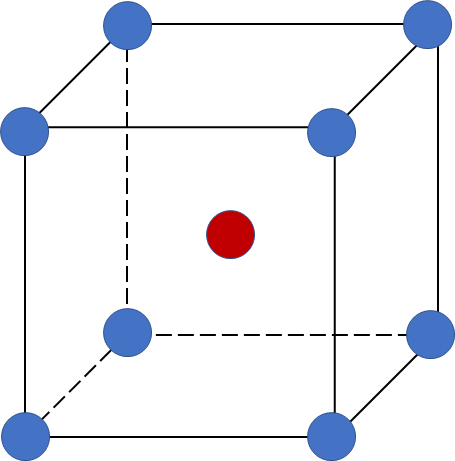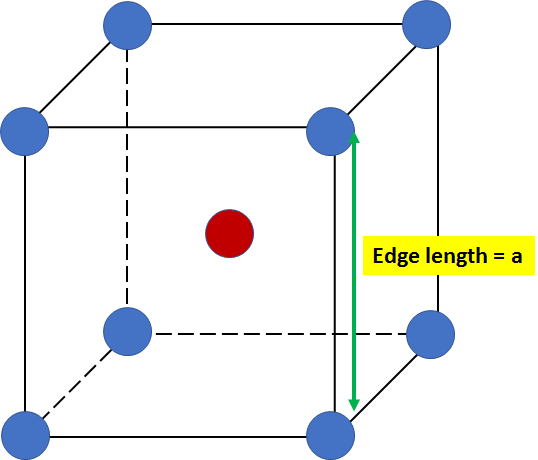# Problem: Iron (Fe) has a BCC crystal structure, an atomic radius of 0.124 nm, and an atomic weight of 55.85 g/mol. What is its theoretical density in g/cm3?

###### FREE Expert Solution

We’re being asked to calculate the density of Fe that crystallizes in a body-centered cubic unit cell.

A body-centered cubic (BCC) unit cell is composed of a cube with one atom at each of its corners and one atom at the center of the cubeRecall that density has a formula of:

$\overline{){\mathbf{density}}{\mathbf{=}}\frac{\mathbf{mass}}{\mathbf{volume}}}$

mass = g
volume = cm3

We’re going to calculate for the density of Fe using the following steps:

Step 1:Calculate the edge length from the atomic radius
Step 2: Calculate the volume of the 1 unit cell
Step 3: Calculate the density of the Fe

Step 1: Calculate the edge length from the atomic radius

a= 2.86x10-8 cm

Calculate the volume of the unit cell.

In a BCC unit cell:Given: edge length (a) = 5.025 Å

volume of unit cell = 2.35 x 10-23 cm3

Step 3: Calculate the density of the Fe###### Problem Details

Iron (Fe) has a BCC crystal structure, an atomic radius of 0.124 nm, and an atomic weight of 55.85 g/mol. What is its theoretical density in g/cm3?